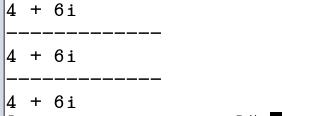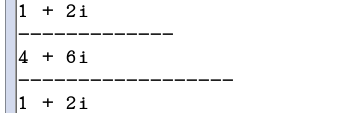• 成员函数和全局函数的对比：  1.类成员函数和全局函数的区别就是，一个是面向对象，一个是面向过程  2.类成员函数==（转成）==>全局函数：增加一个参数，增加的这个参数是代替this指针的  3.全局函数==（转...
类成员函数和全局函数的对比：
1.类成员函数和全局函数的区别就是，一个是面向对象，一个是面向过程
2.类成员函数==（转成）==>全局函数：增加一个参数，增加的这个参数是代替this指针的
3.全局函数==（转成）==>类成员函数：减少一个参数，减少的这个参数通过this指针隐藏
4.这个例子是实现面向过程向面向对象转变
具体代码的实现如下：
Test.h
#ifndef __TEST_H__
#define __TEST_H__

class Test
{
public:
Test(int a, int b)
{
this->a = a;
this->b = b;
}
int getA()
{
return this->a;
}
int getB()
{
return this->b;
}

public:
Test add(Test &t);

private:
int a;
int b;
};

#endif // __TEST_H__main.cpp#include <iostream>
#include "Test.h"
using namespace std;

// 类成员函数：只有一个参数，可以实现两个对象的成员属性相加
Test Test::add(Test &t)
{
Test tmp(this->a + t.getA(), this->b + t.getB());
// 这里能够返回tmp的值，原理是匿名对象实现的
return tmp;
}

// 全局函数：有两个参数，实现两个对象的成员属性相加
Test add(Test &t1, Test &t2)
{
Test tmp(t1.getA() + t2.getA(), t1.getB() + t2.getB());
// 这里能够返回tmp的值，原理是匿名对象实现的
return tmp;
}

int main()
{
// 创建两个类的实例
Test t1(1, 2);
Test t2(3, 4);

// 通过调用类成员函数，返回一个类的实例
Test t3 = t1.add(t2);
cout << t3.getA() << "  " << t3.getB() << endl;
// 通过调用全局函数，返回一个类的实例
Test t4 = add(t1, t2);
cout << t4.getA() << "  " << t4.getB() << endl;

cout << "func main end" << endl;
cin.get();
return 0;
}


展开全文面向过程 面向对象 C++类
• 这段代码的关键在于了解成员函数和全局函数调用的区别 #include <iostream> using namespace std; class Cube{ public: void setM_H(int h) { m_H = h; } int getM_H(){ return m_H; } void setM_L...
这段代码的关键在于了解成员函数和全局函数调用的区别

#include <iostream>
using namespace std;
class Cube{
public:
void setM_H(int h) {
m_H = h;
}
int getM_H(){
return m_H;
}
void setM_L(int l) {
m_L = l;
}
int getM_L() {
return m_L;
}
void setM_W(int w) {
m_W = w;
}
int getM_W() {
return m_W;
}
int calculateS() {
return 2 * m_H * m_L + 2 * m_H * m_W + 2 * m_L * m_W;
}
int calculateV() {
return m_H * m_L * m_W;
}
bool isSameByClass(Cube& c) {
if (m_H == c.getM_H() && m_L == c.getM_L() && m_W == c.getM_W()) {
return true;
}
else {
return false;
}
}
private:
int m_H;
int m_L;
int m_W;
};
//利用全局函数判断是否相等
bool isSame(Cube &c1, Cube &c2) {
if (c1.getM_H() == c2.getM_H() && c1.getM_L() == c2.getM_L() && c1.getM_W() == c2.getM_W()) {
return true;
}
else {
return false;
}
}
int main()
{
Cube c1;
c1.setM_H(10);
c1.setM_L(10);
c1.setM_W(10);
Cube c2;
c2.setM_H(10);
c2.setM_L(10);
c2.setM_W(10);
cout << c1.calculateS() << endl;
cout << c1.calculateV() << endl;
bool ret = isSame(c1, c2);
if (ret) {
cout << "true" << endl;
}
else {
cout << "false" << endl;
}
//利用成员函数判断是否相等
ret = c1.isSameByClass(c2);//用c1调用成员函数在成员函数内传入c2
if (ret) {
cout << "true" << endl;
}
else {
cout << "false" << endl;
}
system("pause");




展开全文• 分别用全局函数和成员函数进行判断两个立方体是否相同*/ class Cube { public: //设置长 void set_L(int l) { L=l; } //获取长 int get_L() { return L; } //设置宽 void set_W(int w) { W = w; ...
#include<iostream>
using namespace std;
/*
设计一个立方体的类，求出立方体的面积和体积
分别用全局函数和成员函数进行判断两个立方体是否相同*/
class Cube
{
public:
//设置长
void set_L(int l)
{
L=l;
}
//获取长
int get_L()
{
return L;
}
//设置宽
void set_W(int w)
{
W = w;
}
//获取宽
int get_W()
{
return W;
}
//设置高
void set_H(int h)
{
H= h;
}
//获取长
int get_H()
{
return H;
}
//计算面积
int S()
{
return L * W * 2 + L * H * 2 + W * H * 2;
}
//计算体积
int V()
{
return L * W * H;
}
//成员函数
bool panduan(Cube &cube)
{
if (L = cube.get_L() && W == cube.get_W() && H == cube.get_H())
{
return true;
}
return false;
}
private:
int L;
int W;
int H;

};
//全局函数
bool Panduan(Cube &cube1,Cube &cube2)
{
if (cube1.get_L() == cube2.get_L()&& cube1.get_W() == cube2.get_W()&& cube1.get_H() == cube2.get_H())
{
return true;
}
return false;
}
int main()
{
Cube cube1;
cube1.set_H (10);
cube1.set_L(10);
cube1.set_W(10);

Cube cube2;
cube2.set_H(100);
cube2.set_L(10);
cube2.set_W(10);
bool t=Panduan(cube1, cube2);
bool p = cube1.panduan(cube2);
if (t == 0&&p==0)
{
cout << "两个立方体不相等" << endl;
}
else
{
cout << "两个立方体相等" << endl;
}
system("pause");
return 0;
}




展开全文• 1、首先，我们要明确成员函数全局函数的区别 ***简单来说，全局变量可以在整个程序里使用；而局部变量只可以在一个函数或一段代码中使用。 （1）类成员函数是面向对象，全局函数是面向过程 （2）类成员函数 =&...
1、首先，我们要明确成员函数与全局函数的区别

***简单来说，全局变量可以在整个程序里使用；而局部变量只可以在一个函数或一段代码中使用。

（1）类成员函数是面向对象，全局函数是面向过程

（2）类成员函数 => 全局函数：增加一个参数，增加的这个参数代替this指针

（3）全局函数 => 类成员函数：减少一个参数，减少的这个参数由this指针隐藏2、其次，明确运算符重载的概念

***重载，就是赋予新的含义。函数重载可以让一个函数名有多重功能，在不同情况下进行不同的操作。

格式如下：

返回值类型 operator 运算符名称 (形参表列)

{
}

例如：Complex operator +(Complex &c)；   //Complex是返回值类型 ；‘+’是运算符；括号内是形参列表

注意：部分运算符不能重载

成员选择符.          域解析运算符::            对象选择符.*             条件运算符: ？            长度运算符sizeof

3、利用成员函数实现运算符的重载

*在这里实现 ‘+’ 运算符和 ‘<<’ 运算符的重载。

值得注意的是，‘+’ 用全局函数或成员函数都能实现重载；但 ‘<<’ 只能用全局函数实现重载。

#include<iostream>
using namespace std;

class Complex
{
friend Complex operator +(Complex &c1 , Complex &c2);    //友元函数
friend ostream &operator <<(ostream &out, Complex &c);
private:
int m_a;
int m_b;
public:
Complex(int a,int b);         //有参构造函数
Complex();                    //无参构造函数
void print();                 //输出复数函数
};

Complex::Complex()
{
m_a = 1;
m_b = 1;
}

Complex::Complex(int a,int b)
{
m_a=a;
m_b=b;
}

//利用全局函数来重载 +
Complex operator +(Complex &c1 , Complex &c2)
{
Complex tmp;          //不能返回引用 只能返回对象

tmp.m_a = c1.m_a + c2.m_a;       //不能直接用私有成员变量 使用友元函数
tmp.m_b = c1.m_b + c2.m_b;

return tmp;
}

//利用全局函数来重载 <<
ostream &operator <<(ostream &out, Complex &c)    //全局函数实现<<重载  一定返回引用
{
out<< c.m_a << "+" <<c.m_b << "i";
return out;
}

void Complex::print()
{
cout<< m_a << " + " << m_b << "i" <<endl;
}

int main()
{
Complex c1(1,2);
Complex c2(3,4);
Complex c3;

operator +(c1,c2);                //与下面的 c1+c2 等价
( operator +(c1,c2) ).print();
cout<< "-------------" <<endl;

c1+c2;
(c1+c2).print();
cout<< "-------------" <<endl;

c3 = c1+c2;
c3.print();
cout<< "--------------" <<endl;

return 0;
}



编译结果如下：4、利用成员函数实现运算符的重载

#include<iostream>
using namespace std;

class Complex
{
friend ostream &operator <<(ostream &out,const Complex &c);
private:
int m_a;
int m_b;
public:
Complex(int a,int b);
Complex();
void print();
Complex operator +(Complex &c);          //成员函数
ostream &operator <<(ostream &out);
};

Complex::Complex()
{
m_a = 1;
m_b = 1;
}

Complex::Complex(int a,int b)
{
m_a=a;
m_b=b;
}

//main函数里面需要用到 '<<' 的重载
ostream &operator <<(ostream &out,const Complex &c)    //成员函数
{
out<< c.m_a << " + " <<c.m_b << "i";
return out;
}

//成员函数实现 '+' 重载   比全局函数少一个参数
Complex Complex::operator +(Complex &c)
{
Complex tmp;
tmp.m_a = this->m_a + c.m_a;       //返回对象本身 而不是返回引用 因为 + 不能作为左值
tmp.m_b = this->m_b + c.m_b;       //若保持c1 c2不变 需要放入另一个tmp里
return tmp;

}

/*
//利用成员函数实现 '+' 的重载 ，有两种方式
Complex Complex::operator +(Complex &c)
{
this->m_a = this->m_a + c.m_a;     //返回对象本身 而不是返回引用 因为 + 不能作为左值
this->m_b = this->m_b + c.m_b;     //此时值都存放于c1中
return *this;
}
*/

/*
//因为不能正常编译通过   所以 << 不能通过成员函数重载
ostream &Complex::operator <<(ostream &out)
{
out<< this->m_a << " + " <<this->m_b << "i";
return out;
}
*/

void Complex::print()
{
cout<< m_a << " + " << m_b << "i" <<endl;
}

int main()
{
Complex c1(1,2);
Complex c2(3,4);
Complex c3;

c1.operator + (c2);        //返回c1里面 变量-->c2
c1.print();
cout<< "-------------" <<endl;

c3 = c1+c2;
cout<< c3 <<endl;          //cout一般输出int型、字符串型等，不能输出对象，所以对'<<'重载
cout<< "------------------" <<endl;

/*
//如果用成员函数进行对 << 的重载，就无法编译通过

c3.operator <<( cout);    //这里强制用函数把cout作为参数，仅仅编译这句可以实现输出

cout << c3 <<endl;        //编译这句时，就不能编译通过

cout<< "----------------" <<endl;
*/

c1+c2 = c3;
c1.print();               //在成员函数中利用tmp可以保持c1 c2的初始值不变

return 0;
}



编译结果如下：5、注意看代码里的注释
展开全文运算符重载
• 成员函数和全局函数 先看一段下面的代码： class Test1 { public: int a; int b; public: Test1(int a, int b) { this->a = a; this->b = b; } }; Test1 T_add(Test1 &t1, Test1 &t2) { Test1 t3; t3.a ...
• 1.如果要在全局函数中调用某个类中的成员函数，那么该类的成员函数需要声明为静态的。因为，普通的类的成员函数通常由该类的对象实例来调用，外部没有途径来访问到它；而声明为静态的，外部则可以通过类名范围限定...
• #include using namespace std; class Test1 { public: Test1(int a=0,int b=0) { this->a=a;... //成员函数 Test1& t_add(Test1 &t2) { this->a=this->a + t2.a; this->b=this->b + t2
• //B:操作符重载实现为非类成员函数(全局函数) //对于全局重载操作符，代表左操作数的参数必须被显式指定。  CA operator+(CA& p1, CA& p2) //const表示只能p改变this，不能反过来  {  cout重载操作符 ...c++
• //ok，编译器首先调用number的构造函数将1转换为number，然后调用成员操作符+ 1 + a; //error，因为它所拥有的是number::operator+，需要左操作数是number对象，因此编译器发出一个出错信息 a - 1; //ok，编译器...编译器 java c++ qt thinkphp
• //ok，编译器首先调用number的构造函数将1转换为number，然后调用成员操作符+ 1 + a; //error，因为它所拥有的是number::operator+，需要左操作数是number对象，因此编译器发出一个出错信息 a - 1; //ok，编译器...
• 测试这个功能的初衷是测试boost里面的bind ...其实成员函数和全局函数无非就是差一个this指针参数。给传进去不久也可以调用了。然而并没有那么简单。看了boost的源码表示太长了。没怎么看懂 然后就c语言 汇编
•c++ c语言
• 1、把全局函数转化成成员函数，通过this指针隐藏左操作数 如：全局函数Test Add(Test &t1,Test &t2) 它的成员函数形式Test Add(Test &t2) 成员函数的应用Test K=t1.Add(t2) 2、把成员函数转化为全局函数...
• //Test1(this, int a, int b) .//全局函数形式 Test1(int a=0, int b=0) { this->a = a; this->b = b; } public: int a; int b; public: //成员函数 Test1& T_add(Test1 &t2) { // Tes
• 全局函数和成员函数全局函数转化成成员函数，通过this指针隐藏左操作数 Test add(Test &t1, Test &t2)===》Test add(Test &t2) 把成员函数转换成全局函数，多了一个参数 void printAB()===》void printAB(Test *...c语言 指针 C++
• 1、把全局函数转化成成员函数，通过this指针隐藏左操作数。 　Test add(Test &t1,Test &t2) ==> Test add(Test & t2); 2、把成员函数转化成全局函数，多了一个参数 　vpid printAB() ==> ...
• 1.把全局函数转化成成员函数 通过this指针隐藏左操作数 Test add(Test &t1, Test &t2)===》Test add(Test &t2) 案例：实现 t1 = t1 + t2 class Test { public: Test(int a = 0,...C++
• 1、把全局函数转化成成员函数，通过this指针隐藏左操作数 Test add(Test &t1, Test &t2)===》Test add(Test &t2) 2、把成员函数转换成全局函数，多了一个参数 void printAB()===》void printAB...
• 一、全局函数重载++（前置） ...二、成员函数重载++（前置） Complex & operator++（） { this->a++; this->b++; return *this; } 三、全局函数重载++（后置） Complex operator++(Complex &c1,in
• 文档标题：函数指针(全局函数/类成员函数)函数对象 原作者：ErranLi 更新日期：2007-01-06 02:31:47 浏览次action class c++builder callback 算法
• 1、把全局函数转化成成员函数，通过this指针隐藏左操作数  Testadd(Test &t1, Test &t2)===》Test add( Test&t2)  2、把成员函数转换成全局函数，多了一个参数  voidprintAB()===》void printAB(Test *pthis) ...
• 这里的测试代码主要是帮助自己了解在类中的this指针。 使用的测试代码主要是：1、使用全局函数...2、使用成员函数实现对象的相加；A& member_add(A &ab)； 系统会解释为：A& member_add(A *pobj,A &ab); 即：会增加一对象 指针 class
• 1，运算符函数既可以是成员函数也可以是全局函数。为了提高性能，通常将全局函数定义为友元。成员函数用this指针隐式地获得一个类对象实参（二元运算符的左操作数）。在全局函数调用中，必须显示地列出表示二元...
• C++深度解析 类中的函数重载 -- 全局函数，普通成员函数，静态成员函数（28）       函数重载的回顾 函数重载的本质为相互独立的不同函数 C++中通过函数名函数参数确定函数调用 无法直接通过函数名得到...
• 在CSDN上发帖讨论关于静态函数与全局静态函数的区别时，有网友指出没有全局静态函数之说，既然是全局函数又怎么能是静态的那？关于这个问题，我不想正面回答，static是C++关键字之一，它的作用就是：把有static修饰......